# ComposedFunction¶

class ComposedFunction(*args)

Composed function.

Available constructor:

ComposedFunction(f, g)

The function is the composed function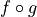.

Parameters:
f,g

The functions to compose.

Examples

>>> import openturns as ot
>>> g = ot.SymbolicFunction(['x1', 'x2'],
...                           ['x1 + x2','3 * x1 * x2'])
>>> f = ot.SymbolicFunction(['x1', 'x2'], ['2 * x1 - x2'])
>>> composed = ot.ComposedFunction(f, g)
>>> print(composed([3, 4]))
[-22]


Methods

 __call__(*args) Call self as a function. draw(*args) Draw the output of function as a Graph. Accessor to the number of times the function has been called. Accessor to the object's name. Accessor to the description of the inputs and outputs. Accessor to the evaluation function. Accessor to the number of times the function has been called. Accessor to the gradient function. Accessor to the number of times the gradient of the function has been called. Accessor to the hessian function. Accessor to the number of times the hessian of the function has been called. Accessor to the object's id. Accessor to the description of the input vector. Accessor to the dimension of the input vector. getMarginal(*args) Accessor to marginal. Accessor to the object's name. Accessor to the description of the output vector. Accessor to the number of the outputs. Accessor to the parameter values. Accessor to the parameter description. Accessor to the dimension of the parameter. Accessor to the object's shadowed id. Accessor to the object's visibility state. gradient(inP) Return the Jacobian transposed matrix of the function at a point. Test if the object is named. Test if the object has a distinguishable name. hessian(inP) Return the hessian of the function at a point. Accessor to the linearity of the function. Accessor to the linearity of the function with regard to a specific variable. Accessor to the gradient against the parameter. setDescription(description) Accessor to the description of the inputs and outputs. setEvaluation(evaluation) Accessor to the evaluation function. setGradient(gradient) Accessor to the gradient function. setHessian(hessian) Accessor to the hessian function. setInputDescription(inputDescription) Accessor to the description of the input vector. setName(name) Accessor to the object's name. setOutputDescription(outputDescription) Accessor to the description of the output vector. setParameter(parameter) Accessor to the parameter values. setParameterDescription(description) Accessor to the parameter description. Accessor to the object's shadowed id. setVisibility(visible) Accessor to the object's visibility state.
__init__(*args)
draw(*args)

Draw the output of function as a Graph.

Available usages:

draw(inputMarg, outputMarg, centralPoint, xiMin, xiMax, ptNb)

draw(firstInputMarg, secondInputMarg, outputMarg, centralPoint, xiMin_xjMin, xiMax_xjMax, ptNbs)

draw(xiMin, xiMax, ptNb)

draw(xiMin_xjMin, xiMax_xjMax, ptNbs)

Parameters:
outputMarg, inputMargint,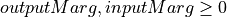outputMarg is the index of the marginal to draw as a function of the marginal with index inputMarg.

firstInputMarg, secondInputMargint,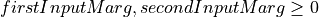In the 2D case, the marginal outputMarg is drawn as a function of the two marginals with indexes firstInputMarg and secondInputMarg.

centralPointsequence of float

Central point with dimension equal to the input dimension of the function.

xiMin, xiMaxfloat

Define the interval where the curve is plotted.

xiMin_xjMin, xiMax_xjMaxsequence of float of dimension 2.

In the 2D case, define the intervals where the curves are plotted.

ptNbint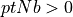or list of ints of dimension 2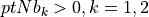The number of points to draw the curves.

Notes

We note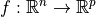where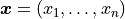and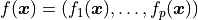, with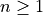and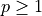.

• In the first usage:

Draws graph of the given 1D outputMarg marginal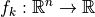as a function of the given 1D inputMarg marginal with respect to the variation of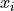in the interval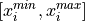, when all the other components of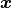are fixed to the corresponding components of the centralPoint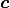. Then OpenTURNS draws the graph: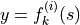for any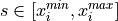where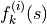is defined by the equation: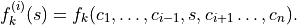• In the second usage:

Draws the iso-curves of the given outputMarg marginal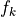as a function of the given 2D firstInputMarg and secondInputMarg marginals with respect to the variation of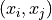in the interval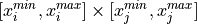, when all the other components ofare fixed to the corresponding components of the centralPoint. Then OpenTURNS draws the graph: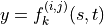for any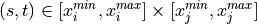where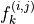is defined by the equation: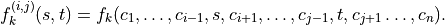• In the third usage:

The same as the first usage but only for function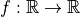.

• In the fourth usage:

The same as the second usage but only for function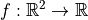.

Examples

>>> import openturns as ot
>>> from openturns.viewer import View
>>> f = ot.SymbolicFunction('x', 'sin(2*pi_*x)*exp(-x^2/2)')
>>> graph = f.draw(-1.2, 1.2, 100)
>>> View(graph).show()

getCallsNumber()

Accessor to the number of times the function has been called.

Returns:
calls_numberint

Integer that counts the number of times the function has been called since its creation.

getClassName()

Accessor to the object’s name.

Returns:
class_namestr

The object class name (object.__class__.__name__).

getDescription()

Accessor to the description of the inputs and outputs.

Returns:
descriptionDescription

Description of the inputs and the outputs.

Examples

>>> import openturns as ot
>>> f = ot.SymbolicFunction(['x1', 'x2'],
...                         ['2 * x1^2 + x1 + 8 * x2 + 4 * cos(x1) * x2 + 6'])
>>> print(f.getDescription())
[x1,x2,y0]

getEvaluation()

Accessor to the evaluation function.

Returns:
functionEvaluationImplementation

The evaluation function.

Examples

>>> import openturns as ot
>>> f = ot.SymbolicFunction(['x1', 'x2'],
...                         ['2 * x1^2 + x1 + 8 * x2 + 4 * cos(x1) * x2 + 6'])
>>> print(f.getEvaluation())
[x1,x2]->[2 * x1^2 + x1 + 8 * x2 + 4 * cos(x1) * x2 + 6]

getEvaluationCallsNumber()

Accessor to the number of times the function has been called.

Returns:
evaluation_calls_numberint

Integer that counts the number of times the function has been called since its creation.

Returns:
gradientGradientImplementation

Accessor to the number of times the gradient of the function has been called.

Returns:

Integer that counts the number of times the gradient of the Function has been called since its creation. Note that if the gradient is implemented by a finite difference method, the gradient calls number is equal to 0 and the different calls are counted in the evaluation calls number.

getHessian()

Accessor to the hessian function.

Returns:
hessianHessianImplementation

The hessian function.

getHessianCallsNumber()

Accessor to the number of times the hessian of the function has been called.

Returns:
hessian_calls_numberint

Integer that counts the number of times the hessian of the Function has been called since its creation. Note that if the hessian is implemented by a finite difference method, the hessian calls number is equal to 0 and the different calls are counted in the evaluation calls number.

getId()

Accessor to the object’s id.

Returns:
idint

Internal unique identifier.

getInputDescription()

Accessor to the description of the input vector.

Returns:
descriptionDescription

Description of the input vector.

Examples

>>> import openturns as ot
>>> f = ot.SymbolicFunction(['x1', 'x2'],
...                          ['2 * x1^2 + x1 + 8 * x2 + 4 * cos(x1) * x2 + 6'])
>>> print(f.getInputDescription())
[x1,x2]

getInputDimension()

Accessor to the dimension of the input vector.

Returns:
inputDimint

Dimension of the input vector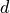.

Examples

>>> import openturns as ot
>>> f = ot.SymbolicFunction(['x1', 'x2'],
...                          ['2 * x1^2 + x1 + 8 * x2 + 4 * cos(x1) * x2 + 6'])
>>> print(f.getInputDimension())
2

getMarginal(*args)

Accessor to marginal.

Parameters:
indicesint or list of ints

Set of indices for which the marginal is extracted.

Returns:
marginalFunction

Function corresponding to either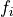or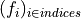, withand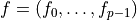.

getName()

Accessor to the object’s name.

Returns:
namestr

The name of the object.

getOutputDescription()

Accessor to the description of the output vector.

Returns:
descriptionDescription

Description of the output vector.

Examples

>>> import openturns as ot
>>> f = ot.SymbolicFunction(['x1', 'x2'],
...                          ['2 * x1^2 + x1 + 8 * x2 + 4 * cos(x1) * x2 + 6'])
>>> print(f.getOutputDescription())
[y0]

getOutputDimension()

Accessor to the number of the outputs.

Returns:
number_outputsint

Dimension of the output vector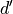.

Examples

>>> import openturns as ot
>>> f = ot.SymbolicFunction(['x1', 'x2'],
...                          ['2 * x1^2 + x1 + 8 * x2 + 4 * cos(x1) * x2 + 6'])
>>> print(f.getOutputDimension())
1

getParameter()

Accessor to the parameter values.

Returns:
parameterPoint

The parameter values.

getParameterDescription()

Accessor to the parameter description.

Returns:
parameterDescription

The parameter description.

getParameterDimension()

Accessor to the dimension of the parameter.

Returns:
parameterDimensionint

Dimension of the parameter.

Accessor to the object’s shadowed id.

Returns:
idint

Internal unique identifier.

getVisibility()

Accessor to the object’s visibility state.

Returns:
visiblebool

Visibility flag.

Return the Jacobian transposed matrix of the function at a point.

Parameters:
pointsequence of float

Point where the Jacobian transposed matrix is calculated.

Returns:
gradientMatrix

The Jacobian transposed matrix of the function at point.

Examples

>>> import openturns as ot
>>> f = ot.SymbolicFunction(['x1', 'x2'],
...                ['2 * x1^2 + x1 + 8 * x2 + 4 * cos(x1) * x2 + 6','x1 + x2'])
[[ 13.5345   1       ]
[  4.00001  1       ]]

hasName()

Test if the object is named.

Returns:
hasNamebool

True if the name is not empty.

hasVisibleName()

Test if the object has a distinguishable name.

Returns:
hasVisibleNamebool

True if the name is not empty and not the default one.

hessian(inP)

Return the hessian of the function at a point.

Parameters:
pointsequence of float

Point where the hessian of the function is calculated.

Returns:
hessianSymmetricTensor

Hessian of the function at point.

Examples

>>> import openturns as ot
>>> f = ot.SymbolicFunction(['x1', 'x2'],
...                ['2 * x1^2 + x1 + 8 * x2 + 4 * cos(x1) * x2 + 6','x1 + x2'])
>>> print(f.hessian([3.14, 4]))
sheet #0
[[ 20          -0.00637061 ]
[ -0.00637061  0          ]]
sheet #1
[[  0           0          ]
[  0           0          ]]

isLinear()

Accessor to the linearity of the function.

Returns:
linearbool

True if the function is linear, False otherwise.

isLinearlyDependent(index)

Accessor to the linearity of the function with regard to a specific variable.

Parameters:
indexint

The index of the variable with regard to which linearity is evaluated.

Returns:
linearbool

True if the function is linearly dependent on the specified variable, False otherwise.

Accessor to the gradient against the parameter.

Returns:
gradientMatrix

setDescription(description)

Accessor to the description of the inputs and outputs.

Parameters:
descriptionsequence of str

Description of the inputs and the outputs.

Examples

>>> import openturns as ot
>>> f = ot.SymbolicFunction(['x1', 'x2'],
...                          ['2 * x1^2 + x1 + 8 * x2 + 4 * cos(x1) * x2 + 6'])
>>> print(f.getDescription())
[x1,x2,y0]
>>> f.setDescription(['a','b','y'])
>>> print(f.getDescription())
[a,b,y]

setEvaluation(evaluation)

Accessor to the evaluation function.

Parameters:
functionEvaluationImplementation

The evaluation function.

Parameters:
gradient_functionGradientImplementation

Examples

>>> import openturns as ot
>>> f = ot.SymbolicFunction(['x1', 'x2'],
...                          ['2 * x1^2 + x1 + 8 * x2 + 4 * cos(x1) * x2 + 6'])
...  f.getEvaluation()))

setHessian(hessian)

Accessor to the hessian function.

Parameters:
hessian_functionHessianImplementation

The hessian function.

Examples

>>> import openturns as ot
>>> f = ot.SymbolicFunction(['x1', 'x2'],
...                         ['2 * x1^2 + x1 + 8 * x2 + 4 * cos(x1) * x2 + 6'])
>>> f.setHessian(ot.CenteredFiniteDifferenceHessian(
...  ot.ResourceMap.GetAsScalar('CenteredFiniteDifferenceHessian-DefaultEpsilon'),
...  f.getEvaluation()))

setInputDescription(inputDescription)

Accessor to the description of the input vector.

Parameters:
descriptionDescription

Description of the input vector.

setName(name)

Accessor to the object’s name.

Parameters:
namestr

The name of the object.

setOutputDescription(outputDescription)

Accessor to the description of the output vector.

Parameters:
descriptionDescription

Description of the output vector.

setParameter(parameter)

Accessor to the parameter values.

Parameters:
parametersequence of float

The parameter values.

setParameterDescription(description)

Accessor to the parameter description.

Parameters:
parameterDescription

The parameter description.

Accessor to the object’s shadowed id.

Parameters:
idint

Internal unique identifier.

setVisibility(visible)

Accessor to the object’s visibility state.

Parameters:
visiblebool

Visibility flag.

## Examples using the class¶Kriging: metamodel of the Branin-Hoo function

Kriging: metamodel of the Branin-Hoo functionKriging : choose a trend vector space

Kriging : choose a trend vector spaceAn illustrated example of a FORM probability estimate

An illustrated example of a FORM probability estimateThe HSIC sensitivity indices: the Ishigami model

The HSIC sensitivity indices: the Ishigami modelCreate a composed function

Create a composed functionIncrease the input dimension of a function

Increase the input dimension of a functionFunction manipulation

Function manipulationSampling from an unnormalized probability density

Sampling from an unnormalized probability density